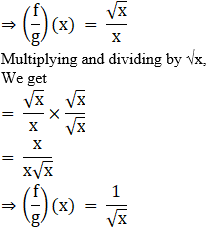# NCERT Exemplar Solutions for Class 11 Maths Chapter 2 Relations and Functions

NCERT Exemplar Solutions for Class 11 Maths Chapter 2 Relations and Functions are created by the subject experts at BYJU’S. These Solutions of NCERT Exemplar Maths help students in solving problems efficiently. They also focus on cracking the solutions of Maths and making it easy for students. NCERT Exemplar Solutions for Class 11 aim at equipping them with detailed, step-wise explanations for all answers to questions given in the exercises of this chapter.

Chapter 2 Relations and Functions of NCERT Exemplar Solutions for Class 11 Maths explains the concepts related to relations and functions. A relation can be defined as a set of outputs as well as inputs. These are also written as ordered pairs by representing a relation of a graph or by a mapping diagram. The graph of the relationship is one of the best visual indicators between the inputs and the outputs. Functions, on the other hand, are the relation with a single input and output. These Exemplar Solutions can also be used as a reference tool while preparing for the final exam. Click here to get exemplar solutions for all chapters. In Chapter 2, students will learn and solve exemplar problems based on topics, like

• Cartesian product of sets
• Relations between sets
• Functions on sets
• Identity function
• Constant function
• Polynomial function
• Rational function
• The Modulus function
• Signum function
• Greatest integer function
• Algebra of functions
• Addition of two real functions
• Subtraction of a real function from another
• Multiplication by a Scalar
• Multiplication of two real functions
• Quotient of two real function
• Graphical representation of functions
• Algebraic representation of real functions

## Download the PDF of the NCERT Exemplar Class 11 Maths Solutions Chapter 2 Relations and Functions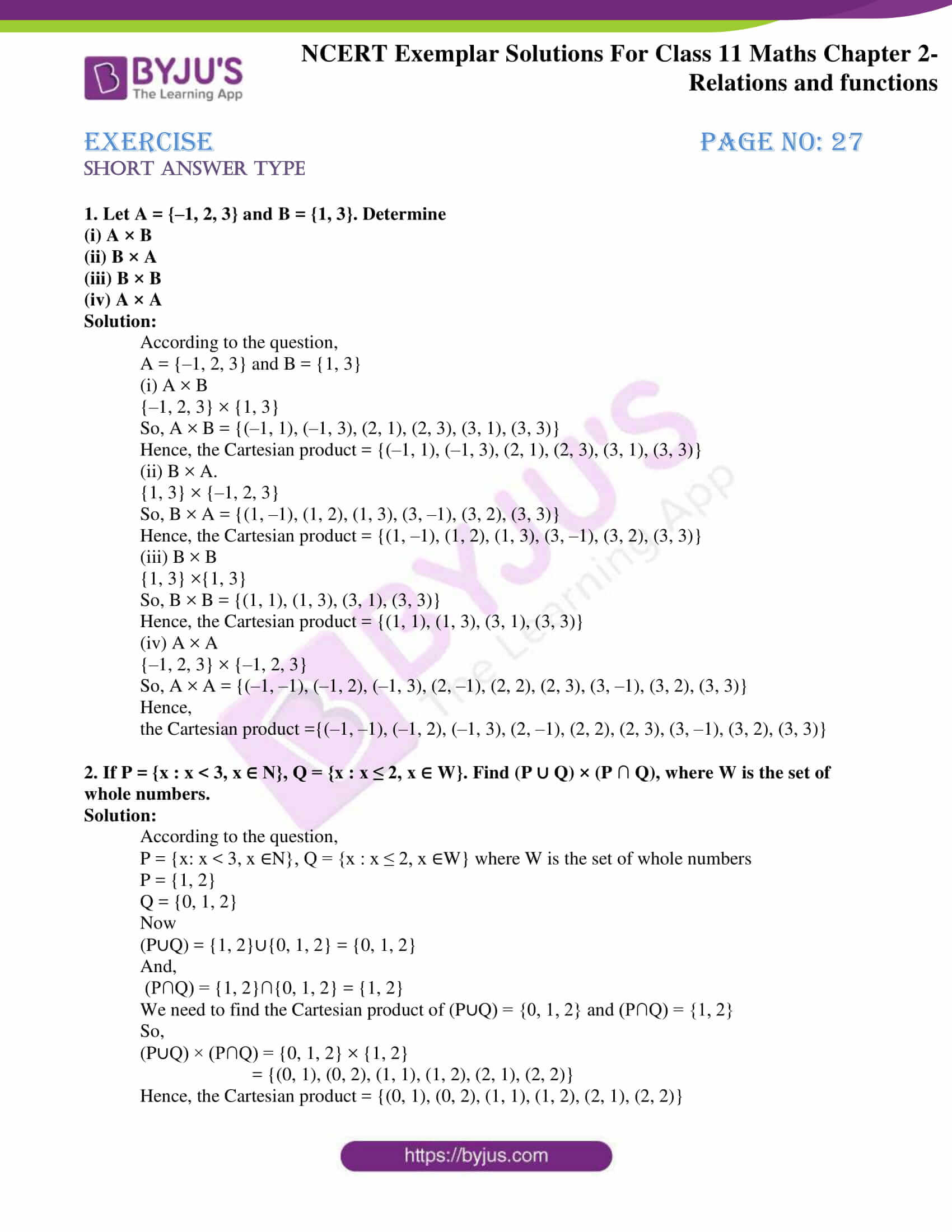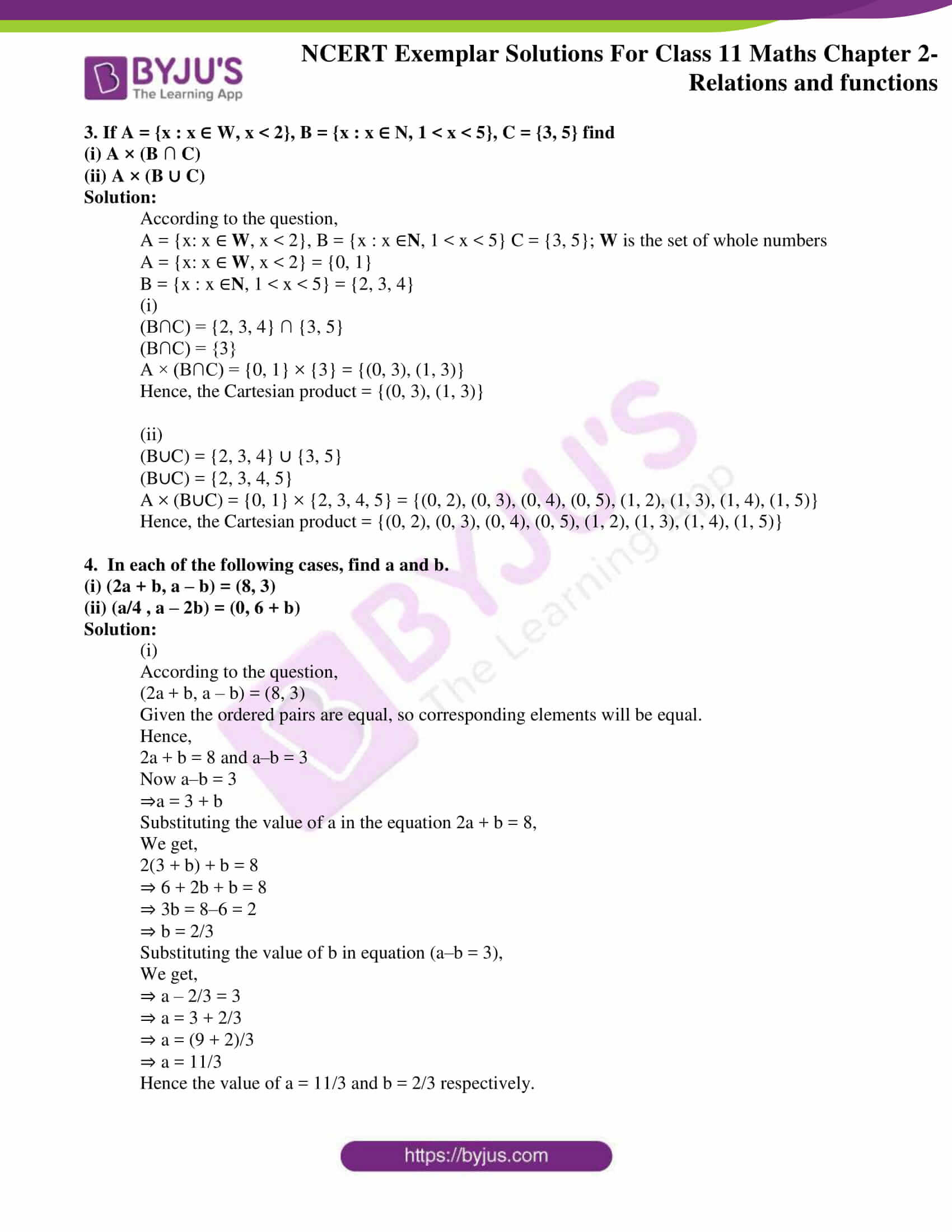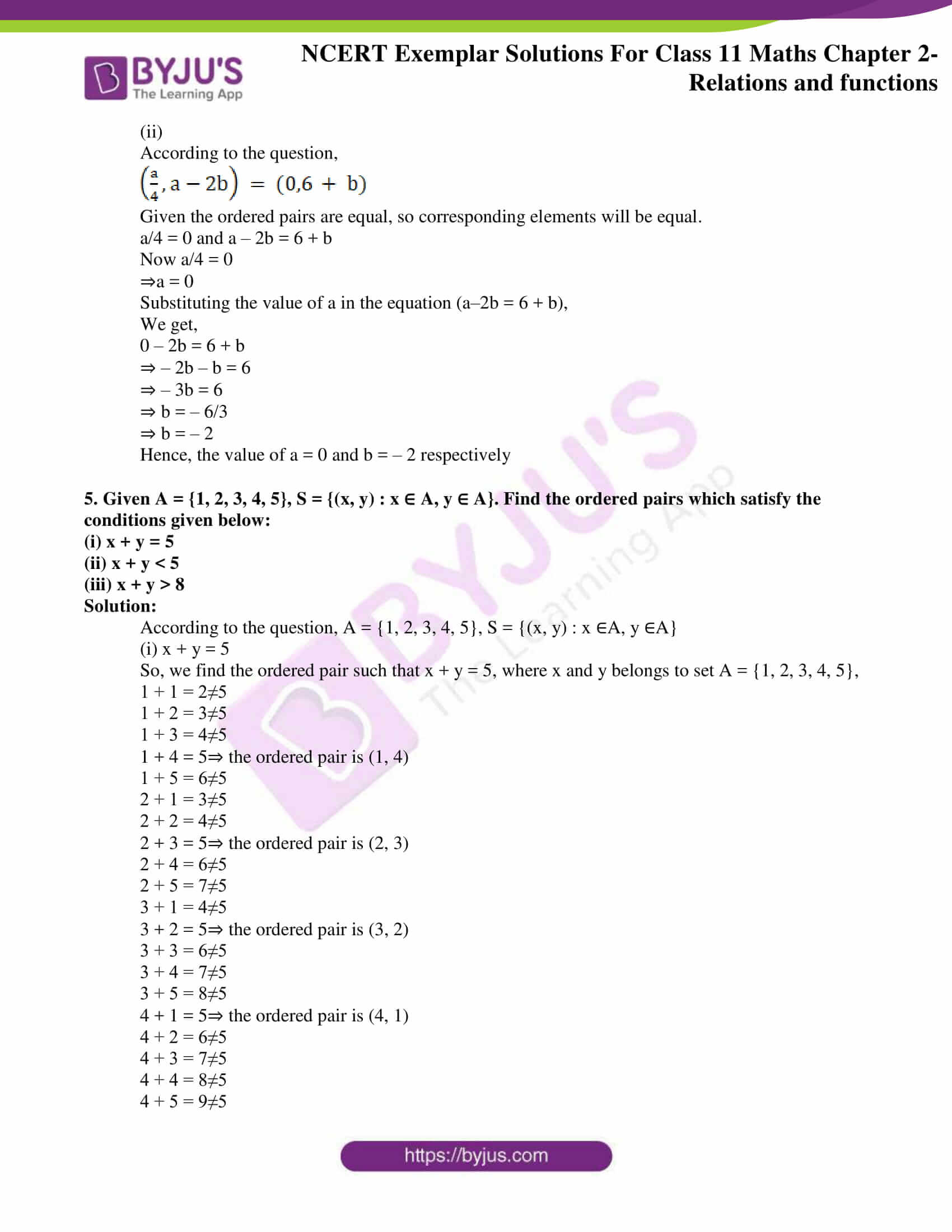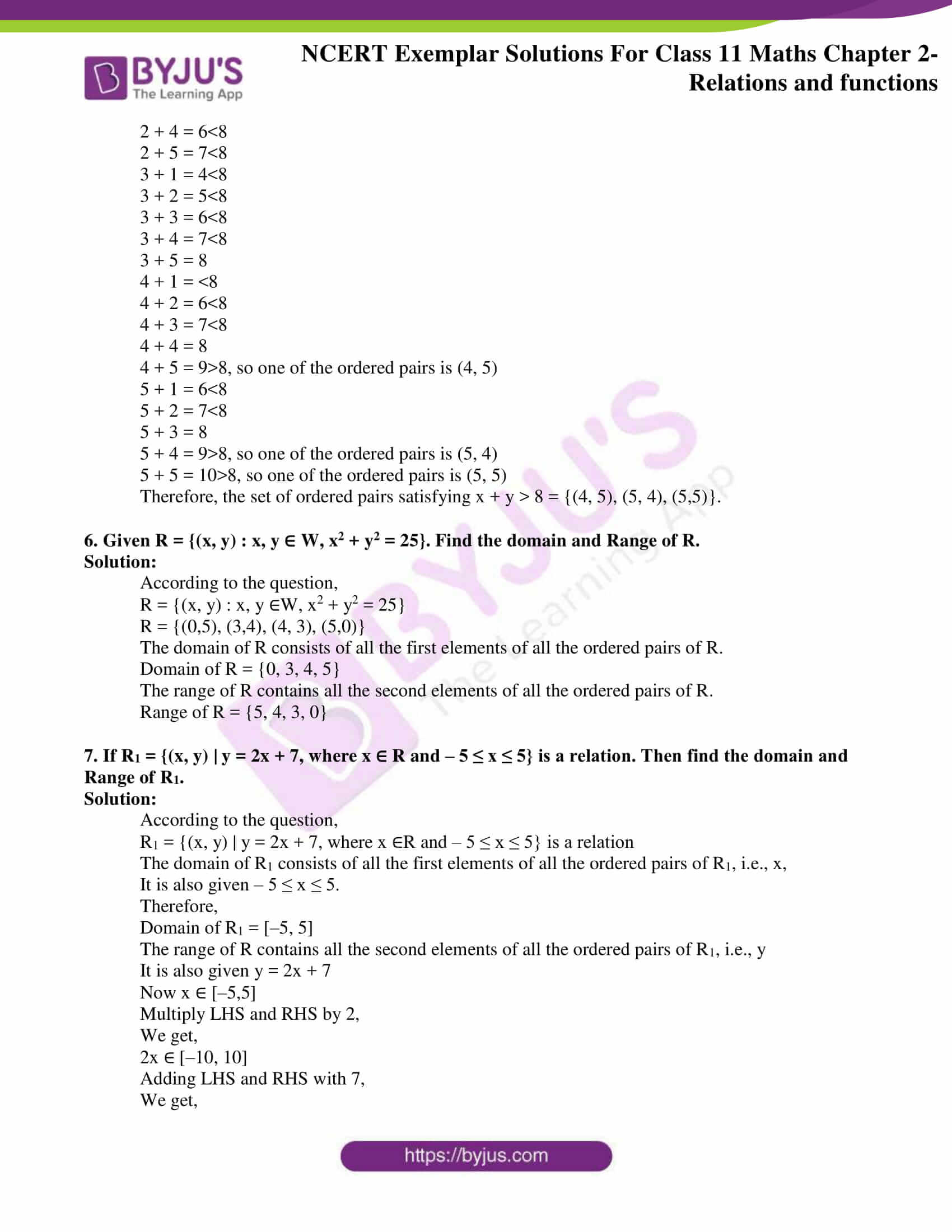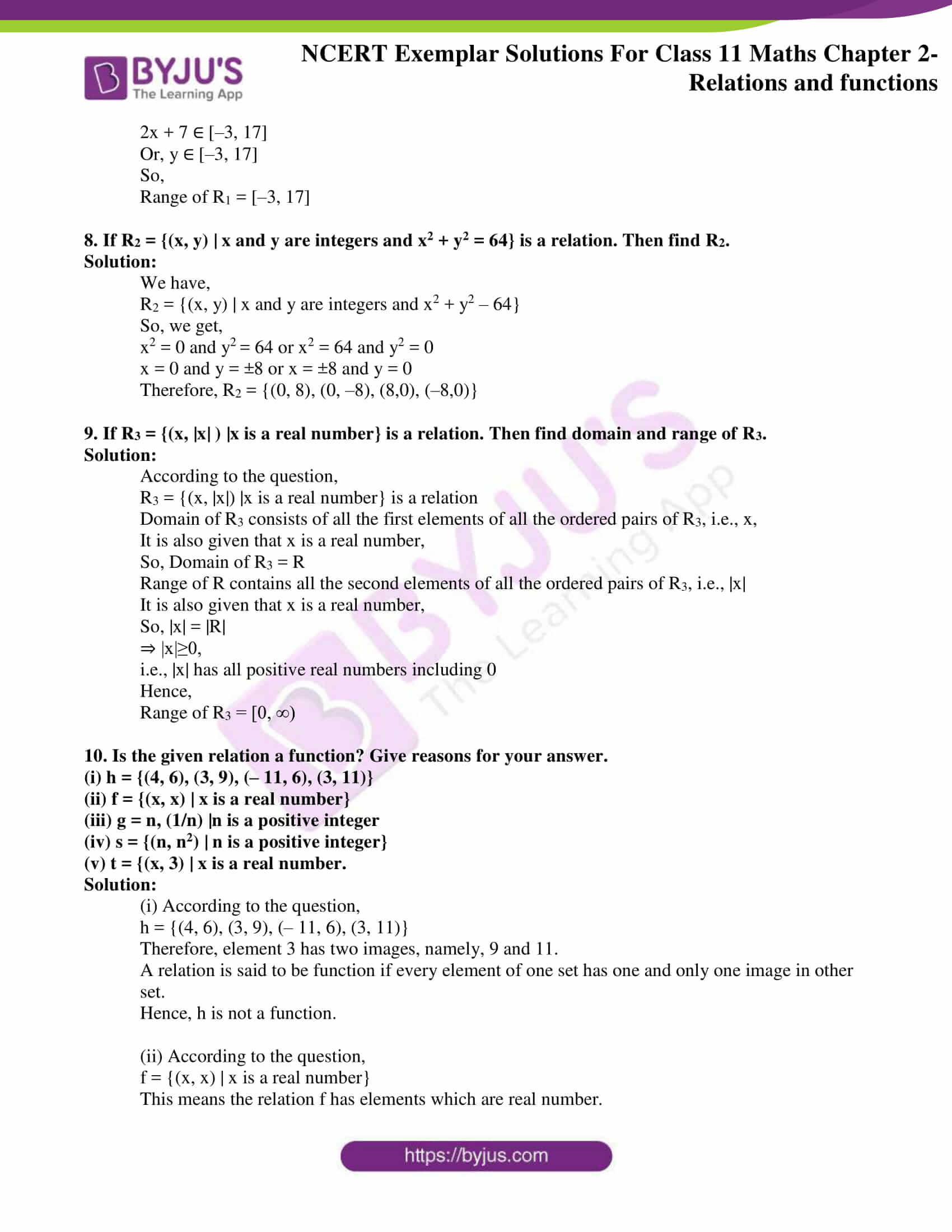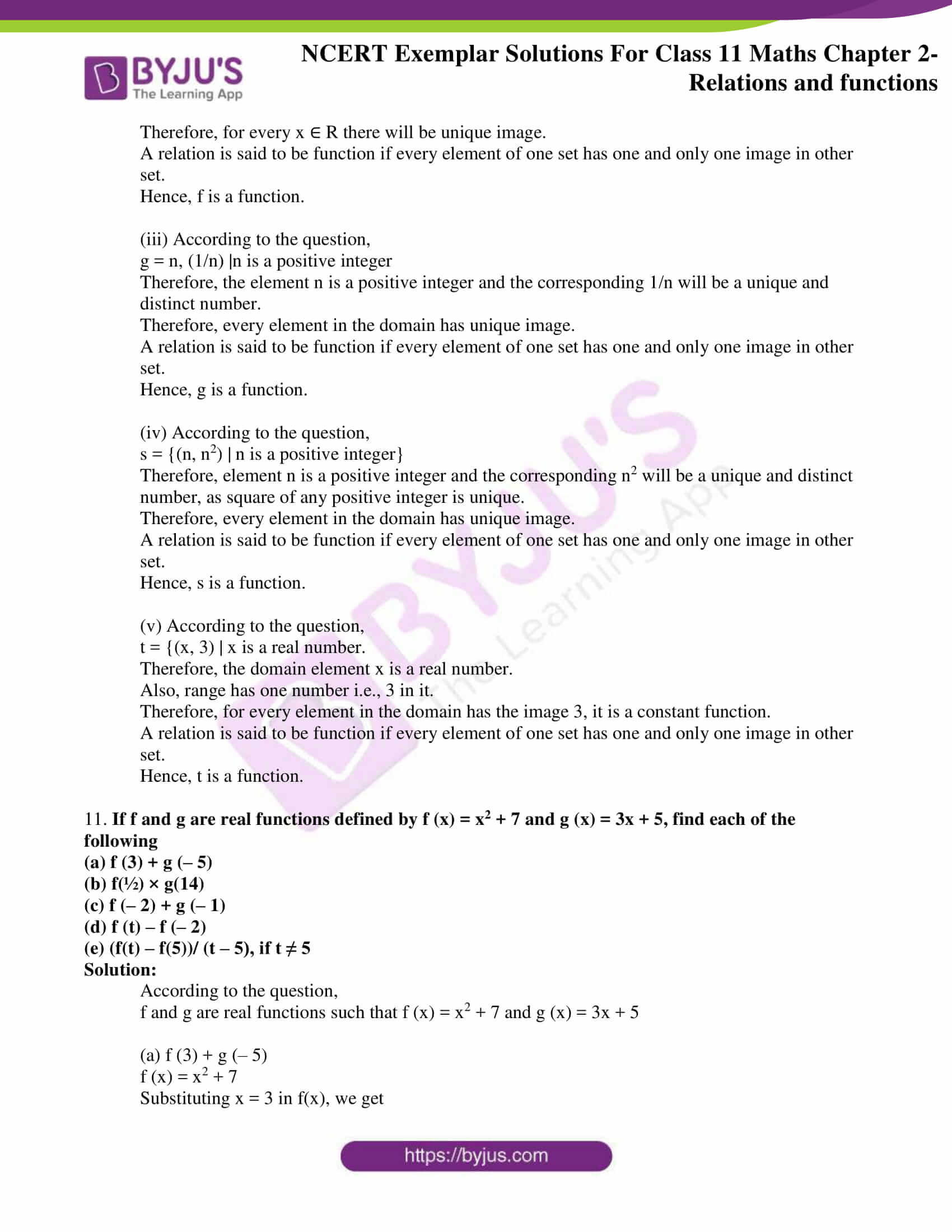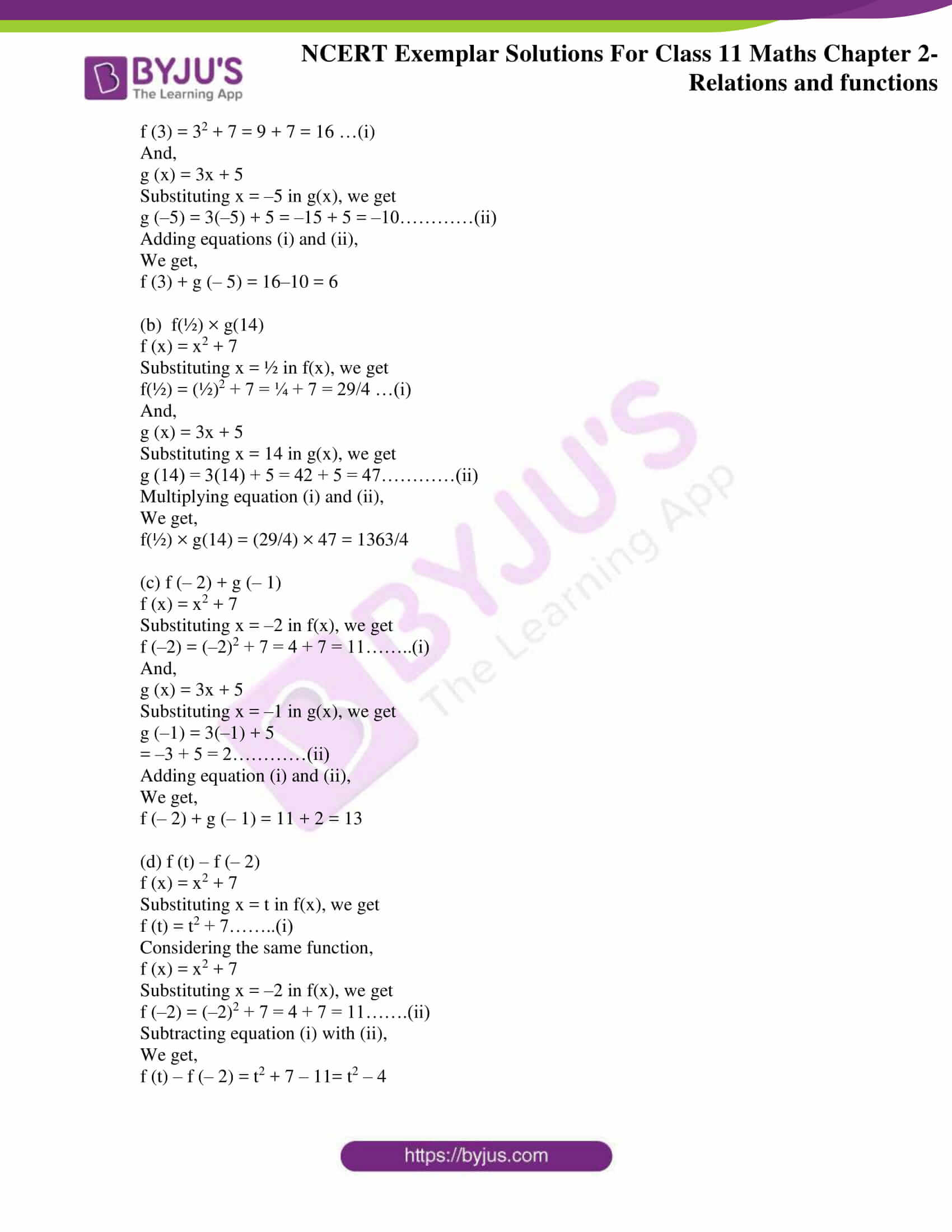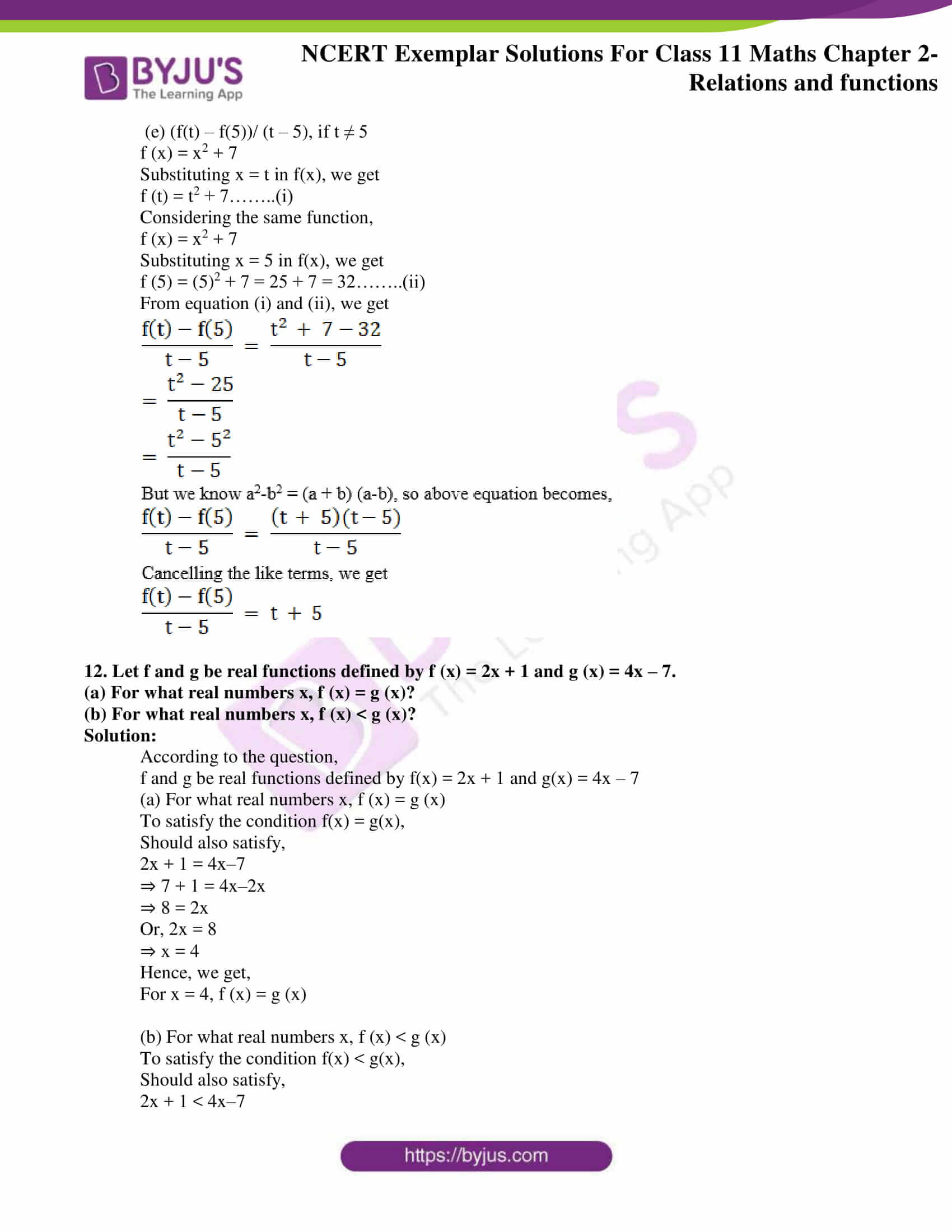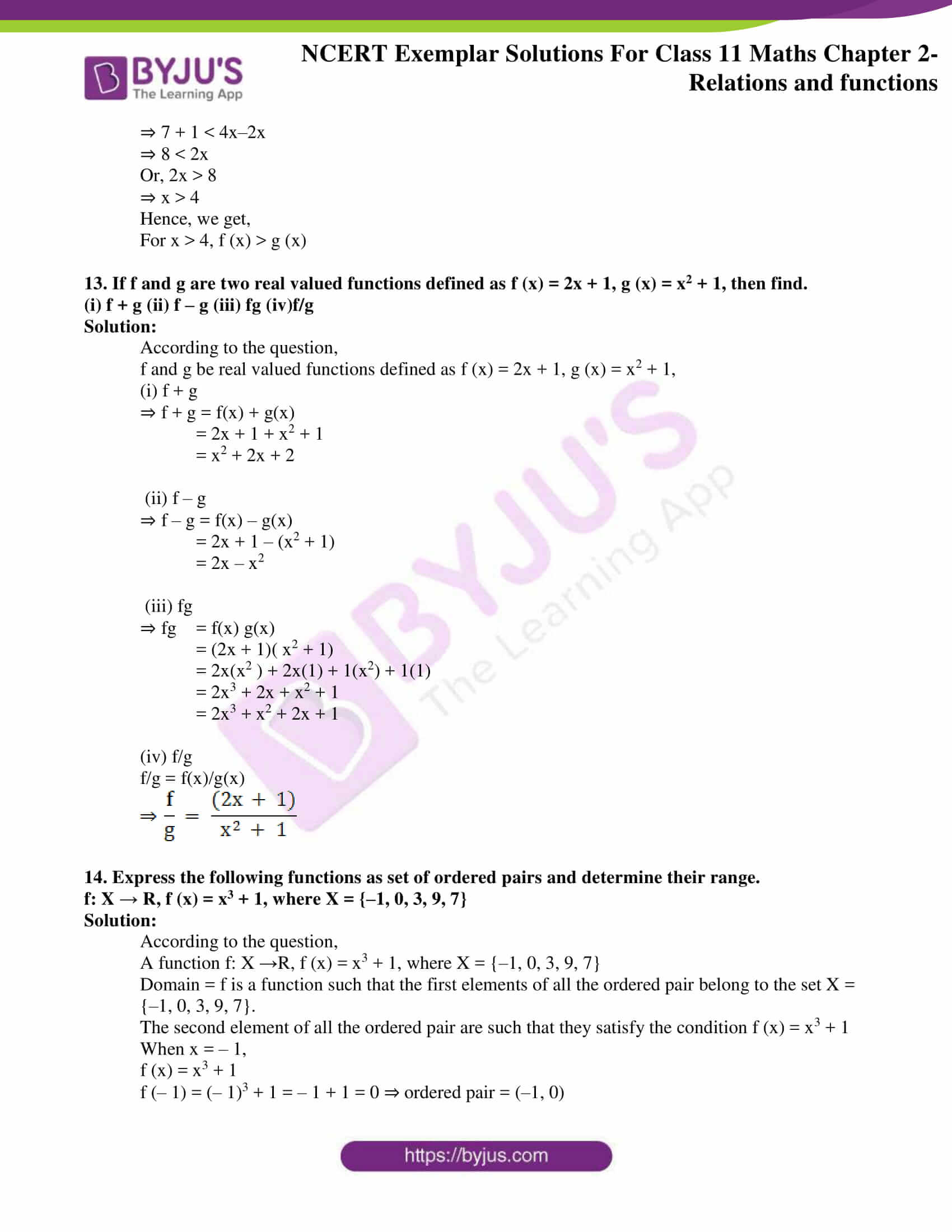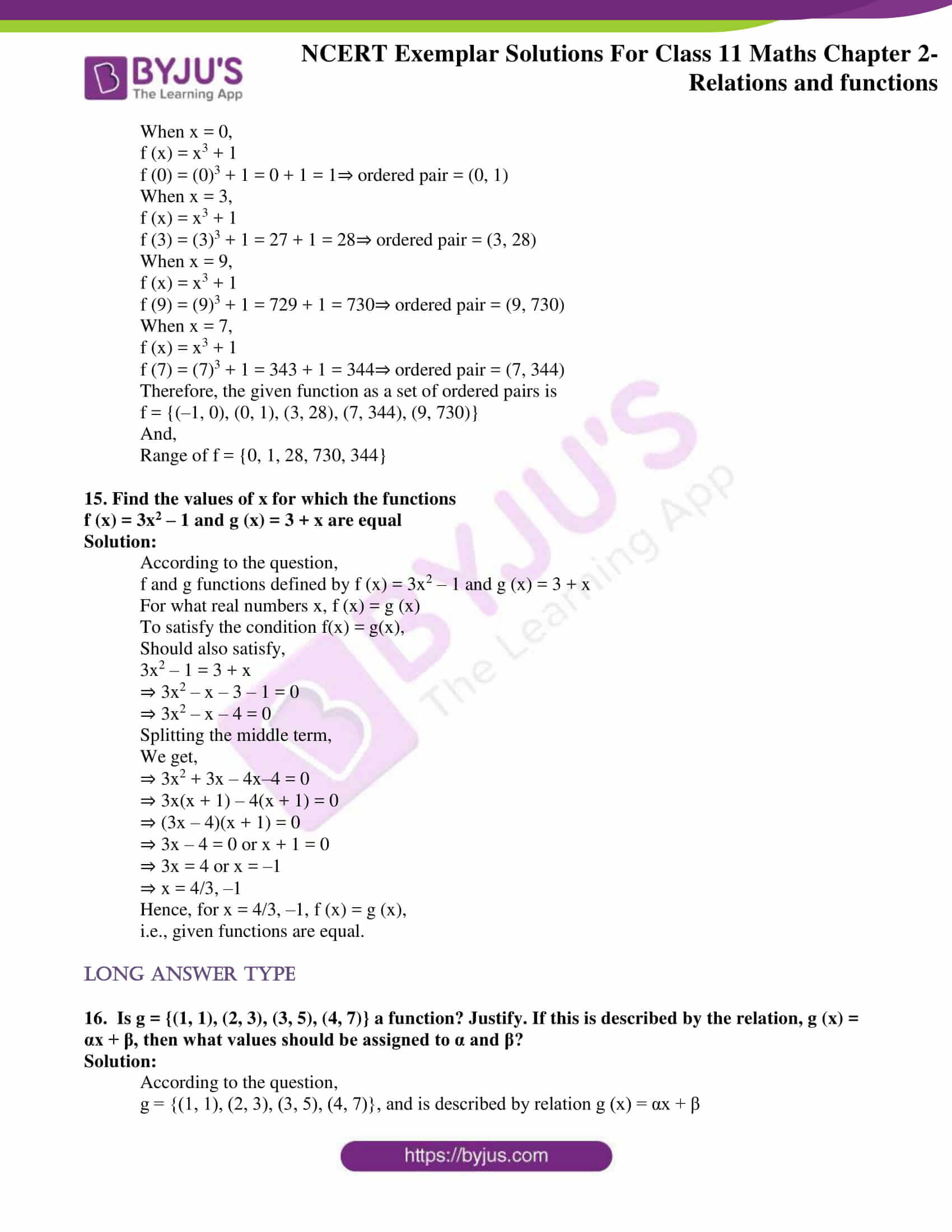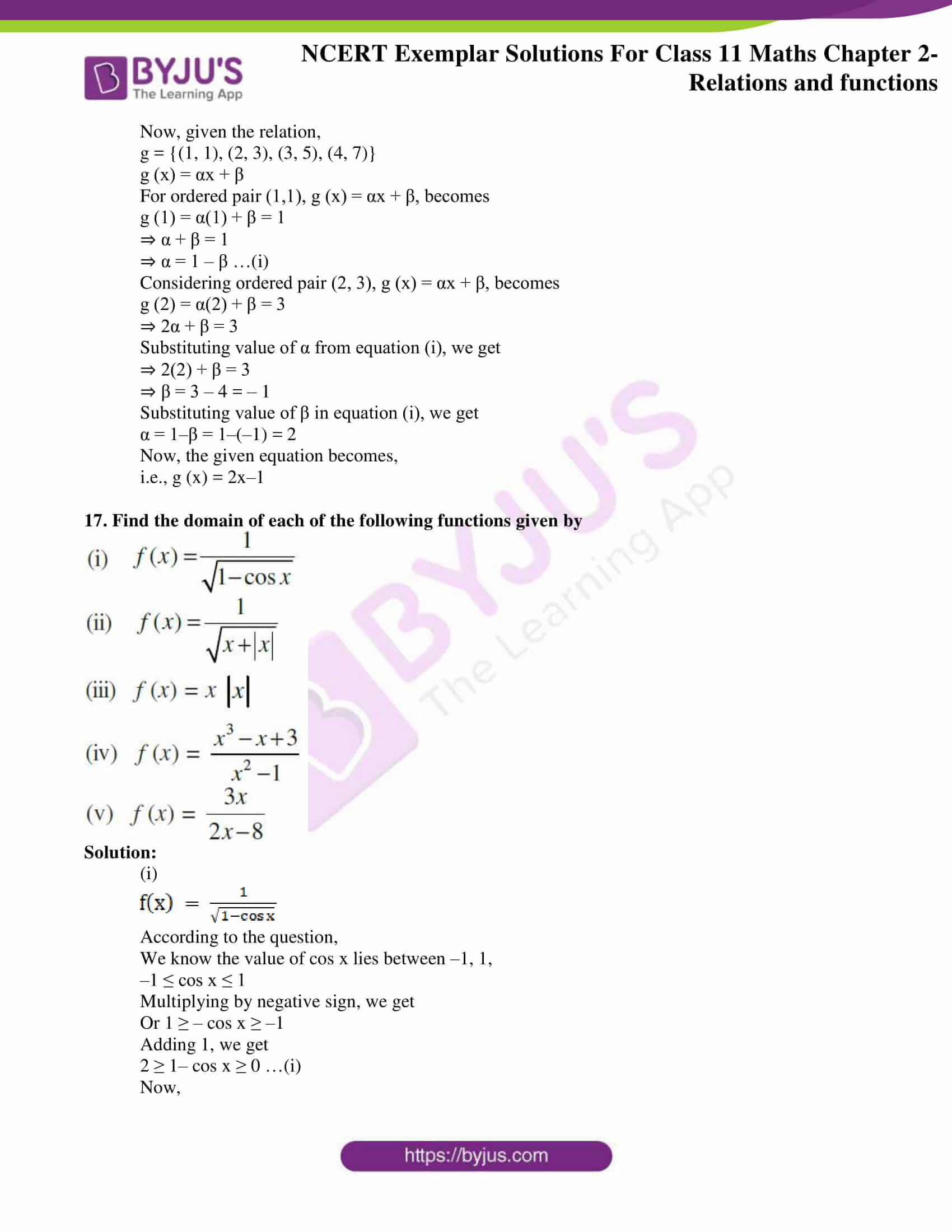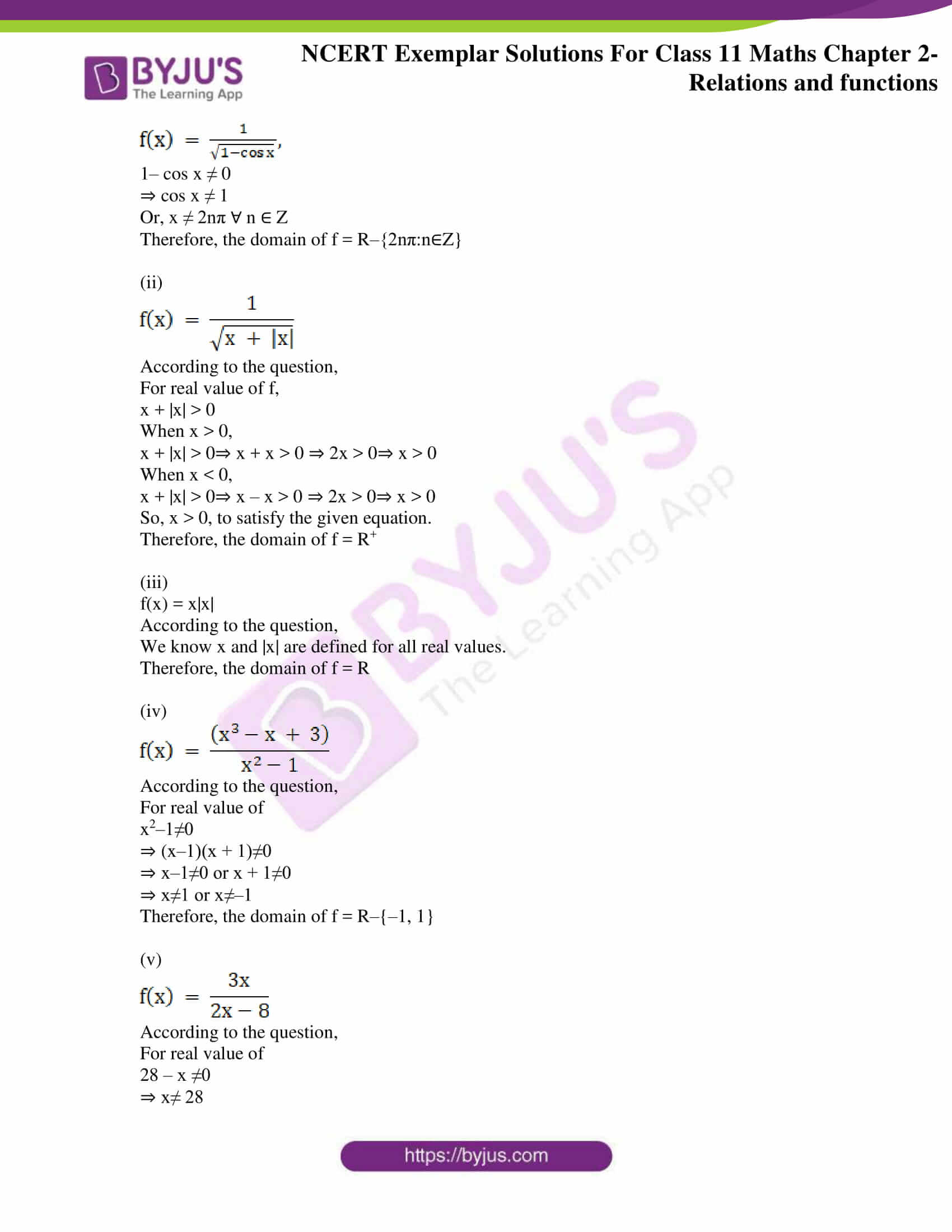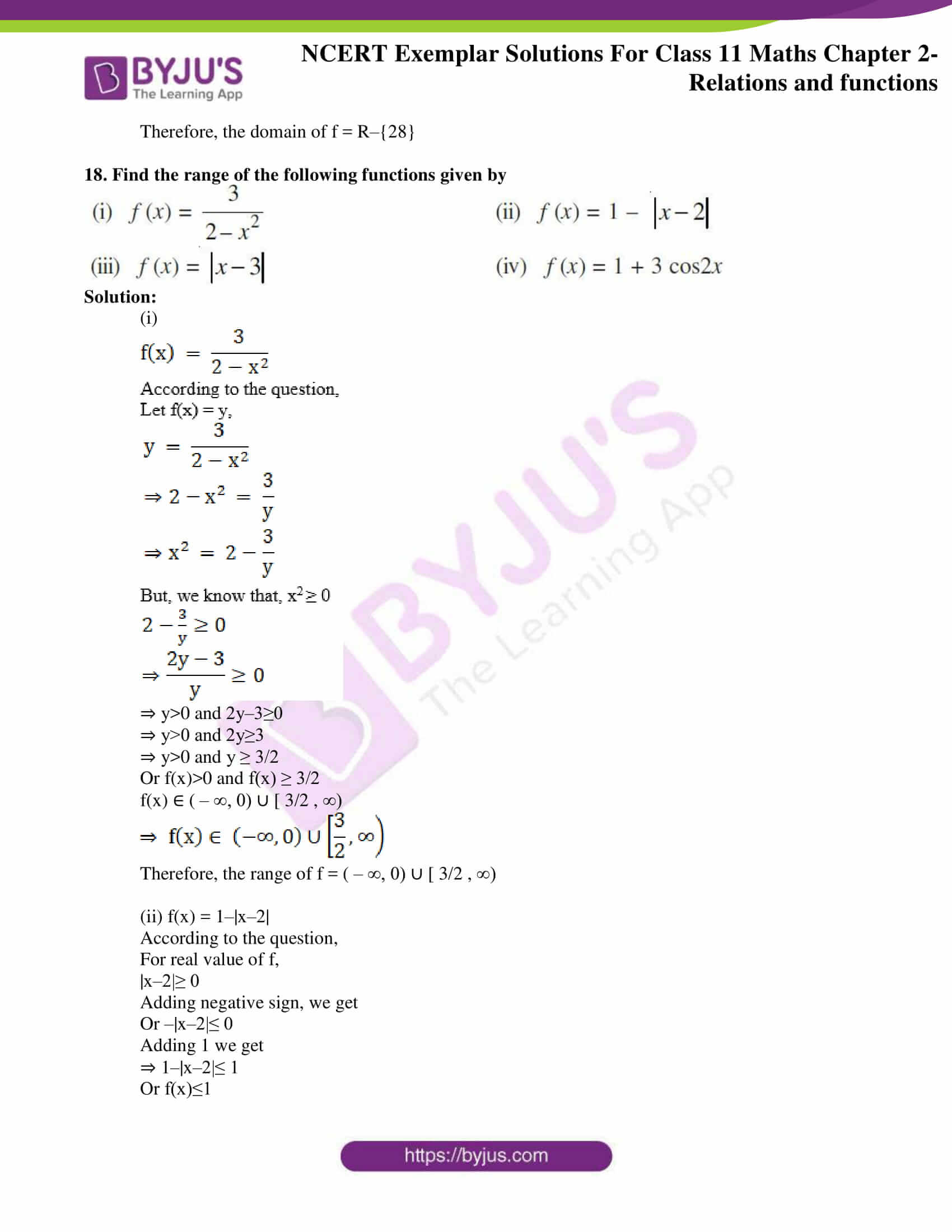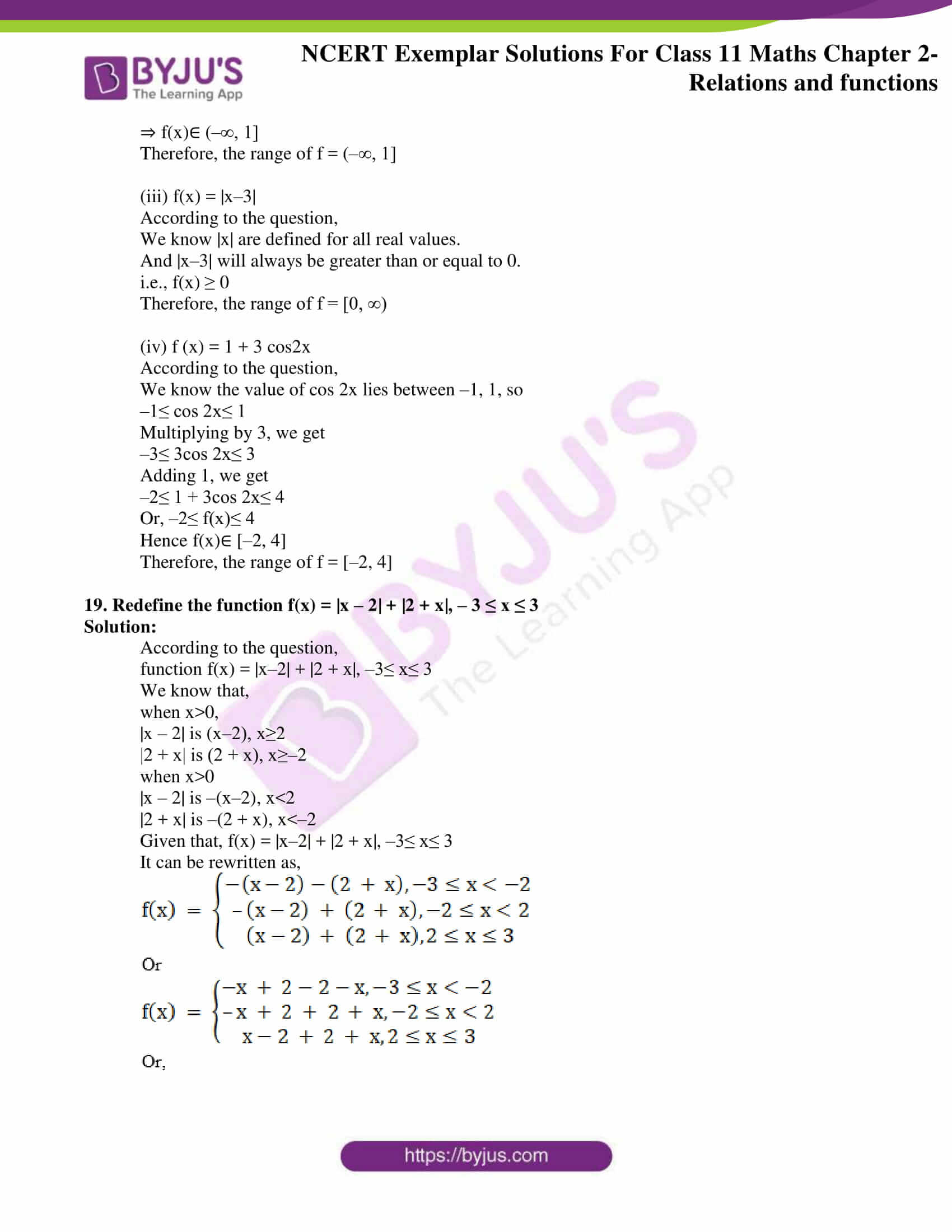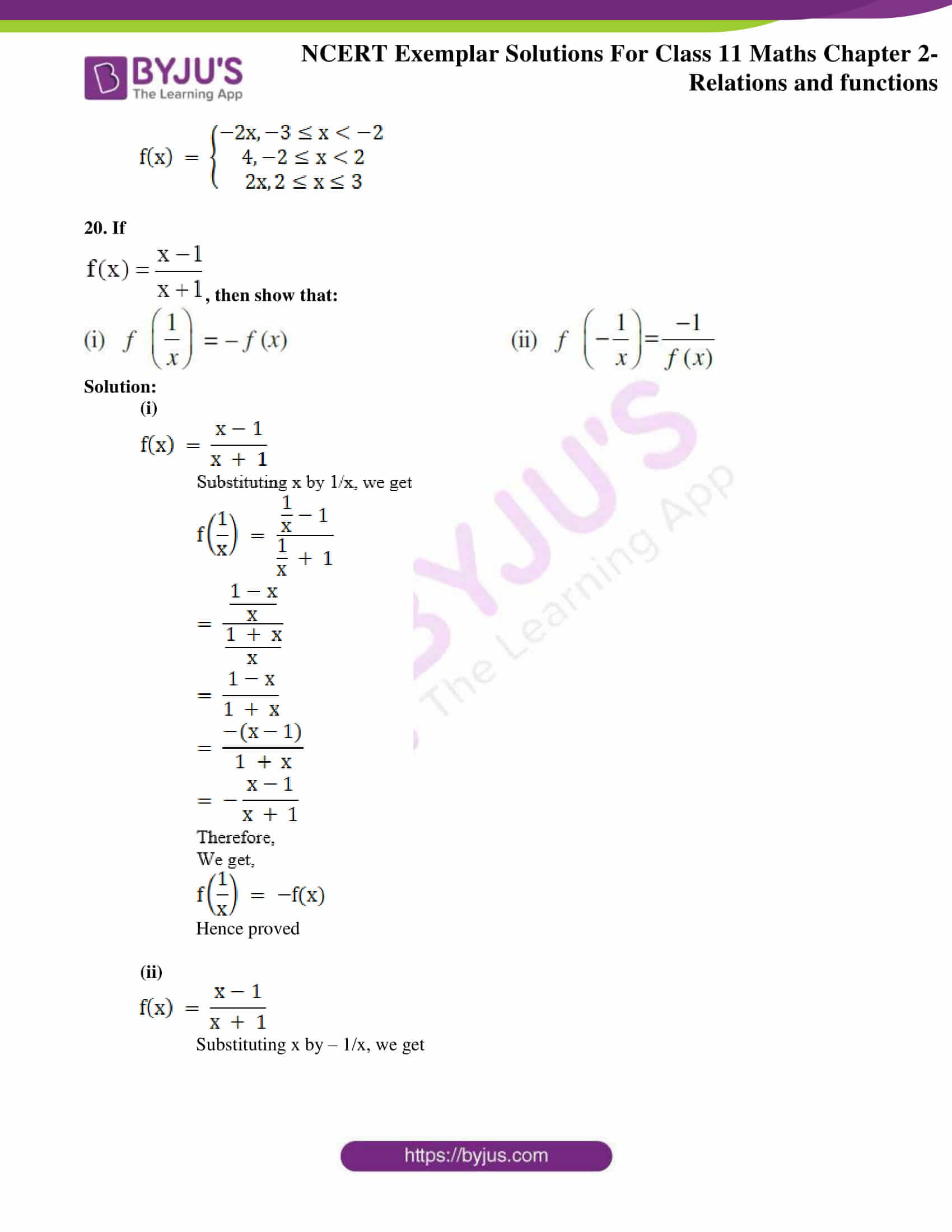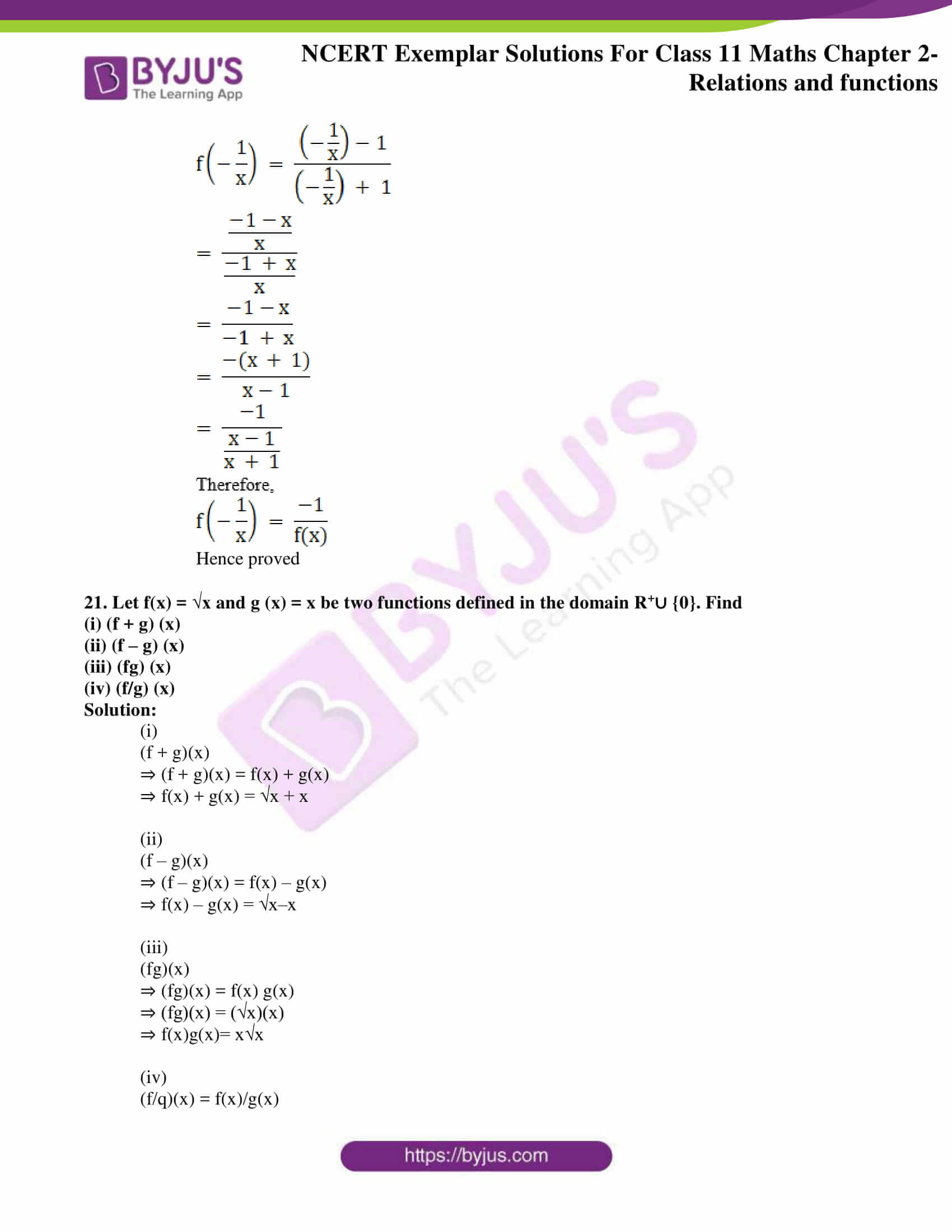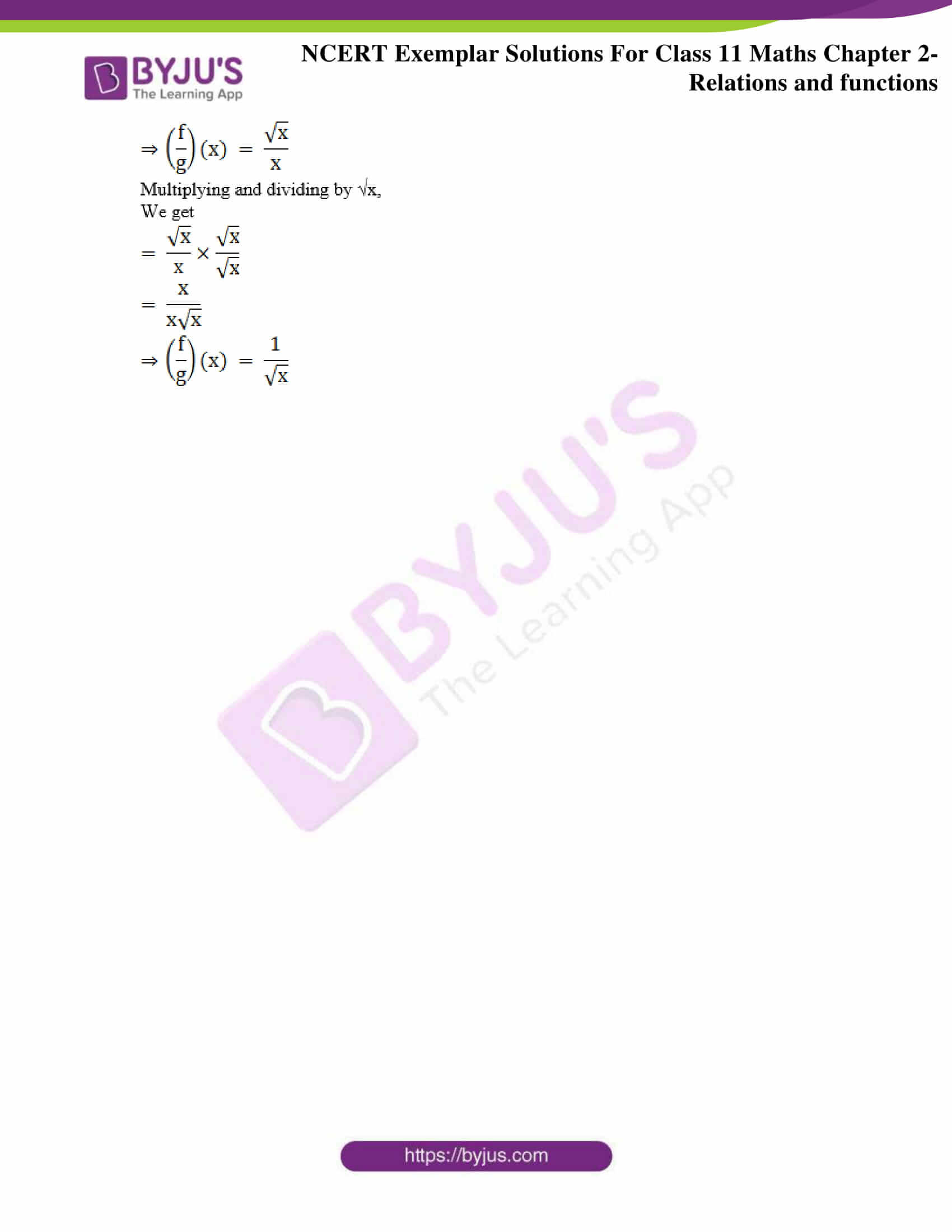### Access Answers to the NCERT Exemplar Class 11 Maths Solutions Chapter 2 Relations and Functions

Exercise Page No: 27

1. Let A = {–1, 2, 3} and B = {1, 3}. Determine
(i) A × B

(ii) B × A
(iii) B × B

(iv) A × A

Solution:

According to the question,

A = {–1, 2, 3} and B = {1, 3}

(i) A × B

{–1, 2, 3} × {1, 3}

So, A × B = {(–1, 1), (–1, 3), (2, 1), (2, 3), (3, 1), (3, 3)}

Hence, the Cartesian product = {(–1, 1), (–1, 3), (2, 1), (2, 3), (3, 1), (3, 3)}

(ii) B × A.

{1, 3} × {–1, 2, 3}

So, B × A = {(1, –1), (1, 2), (1, 3), (3, –1), (3, 2), (3, 3)}

Hence, the Cartesian product = {(1, –1), (1, 2), (1, 3), (3, –1), (3, 2), (3, 3)}

(iii) B × B

{1, 3} ×{1, 3}

So, B × B = {(1, 1), (1, 3), (3, 1), (3, 3)}

Hence, the Cartesian product = {(1, 1), (1, 3), (3, 1), (3, 3)}

(iv) A × A

{–1, 2, 3} × {–1, 2, 3}

So, A × A = {(–1, –1), (–1, 2), (–1, 3), (2, –1), (2, 2), (2, 3), (3, –1), (3, 2), (3, 3)}

Hence,

the Cartesian product ={(–1, –1), (–1, 2), (–1, 3), (2, –1), (2, 2), (2, 3), (3, –1), (3, 2), (3, 3)}

2. If P = {x : x < 3, x ∈ N}, Q = {x : x ≤ 2, x ∈ W}. Find (P ∪ Q) × (P ∩ Q), where W is the set of whole numbers.

Solution:

According to the question,

P = {x: x < 3, x ∈N}, Q = {x : x ≤ 2, x ∈W} where W is the set of whole numbers

P = {1, 2}

Q = {0, 1, 2}

Now

(P∪Q) = {1, 2}∪{0, 1, 2} = {0, 1, 2}

And,

(P∩Q) = {1, 2}∩{0, 1, 2} = {1, 2}

We need to find the Cartesian product of (P∪Q) = {0, 1, 2} and (P∩Q) = {1, 2}

So,

(P∪Q) × (P∩Q) = {0, 1, 2} × {1, 2}

= {(0, 1), (0, 2), (1, 1), (1, 2), (2, 1), (2, 2)}

Hence, the Cartesian product = {(0, 1), (0, 2), (1, 1), (1, 2), (2, 1), (2, 2)}

3. If A = {x : x ∈ W, x < 2}, B = {x : x ∈ N, 1 < x < 5}, C = {3, 5} find
(i) A × (B ∩ C)
(ii) A × (B ∪ C)

Solution:

According to the question,

A = {x: x ∈ W, x < 2}, B = {x : x ∈N, 1 < x < 5} C = {3, 5}; W is the set of whole numbers

A = {x: x ∈ W, x < 2} = {0, 1}

B = {x : x ∈N, 1 < x < 5} = {2, 3, 4}

(i)

(B∩C) = {2, 3, 4} ∩ {3, 5}

(B∩C) = {3}

A × (B∩C) = {0, 1} × {3} = {(0, 3), (1, 3)}

Hence, the Cartesian product = {(0, 3), (1, 3)}

(ii)

(B∪C) = {2, 3, 4} ∪ {3, 5}

(B∪C) = {2, 3, 4, 5}

A × (B∪C) = {0, 1} × {2, 3, 4, 5} = {(0, 2), (0, 3), (0, 4), (0, 5), (1, 2), (1, 3), (1, 4), (1, 5)}

Hence, the Cartesian product = {(0, 2), (0, 3), (0, 4), (0, 5), (1, 2), (1, 3), (1, 4), (1, 5)}

4. In each of the following cases, find a and b.
(i) (2a + b, a – b) = (8, 3)

(ii) (a/4 , a – 2b) = (0, 6 + b)

Solution:

(i)

According to the question,

(2a + b, a – b) = (8, 3)

Given the ordered pairs are equal, so corresponding elements will be equal.

Hence,

2a + b = 8 and a–b = 3

Now a–b = 3

⇒a = 3 + b

Substituting the value of a in the equation 2a + b = 8,

We get,

2(3 + b) + b = 8

⇒ 6 + 2b + b = 8

⇒ 3b = 8–6 = 2

⇒ b = 2/3

Substituting the value of b in equation (a–b = 3),

We get,

⇒ a – 2/3 = 3

⇒ a = 3 + 2/3

⇒ a = (9 + 2)/3

⇒ a = 11/3

Hence the value of a = 11/3 and b = 2/3 respectively.

(ii)

According to the question,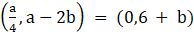Given the ordered pairs are equal, so corresponding elements will be equal.

a/4 = 0 and a – 2b = 6 + b

Now a/4 = 0

⇒a = 0

Substituting the value of a in the equation (a–2b = 6 + b),

We get,

0 – 2b = 6 + b

⇒ – 2b – b = 6

⇒ – 3b = 6

⇒ b = – 6/3

⇒ b = – 2

Hence, the value of a = 0 and b = – 2 respectively

5. Given A = {1, 2, 3, 4, 5}, S = {(x, y) : x ∈ A, y ∈ A}. Find the ordered pairs which satisfy the conditions given below:

(i) x + y = 5

(ii) x + y < 5

(iii) x + y > 8

Solution:

According to the question, A = {1, 2, 3, 4, 5}, S = {(x, y) : x ∈A, y ∈A}

(i) x + y = 5

So, we find the ordered pair such that x + y = 5, where x and y belongs to set A = {1, 2, 3, 4, 5},

1 + 1 = 2≠5

1 + 2 = 3≠5

1 + 3 = 4≠5

1 + 4 = 5⇒ the ordered pair is (1, 4)

1 + 5 = 6≠5

2 + 1 = 3≠5

2 + 2 = 4≠5

2 + 3 = 5⇒ the ordered pair is (2, 3)

2 + 4 = 6≠5

2 + 5 = 7≠5

3 + 1 = 4≠5

3 + 2 = 5⇒ the ordered pair is (3, 2)

3 + 3 = 6≠5

3 + 4 = 7≠5

3 + 5 = 8≠5

4 + 1 = 5⇒ the ordered pair is (4, 1)

4 + 2 = 6≠5

4 + 3 = 7≠5

4 + 4 = 8≠5

4 + 5 = 9≠5

5 + 1 = 6≠5

5 + 2 = 7≠5

5 + 3 = 8≠5

5 + 4 = 9≠5

5 + 5 = 10≠5

Therefore, the set of ordered pairs satisfying x + y = 5 = {(1,4), (2,3), (3,2), (4,1)}.

(ii) x + y < 5

So, we find the ordered pair such that x + y<5, where x and y belongs to set A = {1, 2, 3, 4, 5}

1 + 1 = 2<5 ⇒ the ordered pairs is (1, 1)

1 + 2 = 3<5 ⇒ the ordered pairs is (1, 2)

1 + 3 = 4<5 ⇒ the ordered pairs is (1, 3)

1 + 4 = 5

1 + 5 = 6>5

2 + 1 = 3<5 ⇒ the ordered pairs is (2, 1)

2 + 2 = 4<5 ⇒ the ordered pairs is (2, 2)

2 + 3 = 5

2 + 4 = 6>5

2 + 5 = 7>5

3 + 1 = 4<5 ⇒ the ordered pairs is (3, 1)

3 + 2 = 5

3 + 3 = 6>5

3 + 4 = 7>5

3 + 5 = 8>5

4 + 1 = 5

4 + 2 = 6>5

4 + 3 = 7>5

4 + 4 = 8>5

4 + 5 = 9>5

5 + 1 = 6>5

5 + 2 = 7>5

5 + 3 = 8>5

5 + 4 = 9>5

5 + 5 = 10>5

Therefore, the set of ordered pairs satisfying x + y< 5 = {(1,1), (1,2), (1,3), (2, 1), (2,2), (3,1)}.

(iii) x + y > 8

So, we find the ordered pair such that x + y>8, where x and y belongs to set A = {1, 2, 3, 4, 5}

1 + 1 = 2<8

1 + 2 = 3<8

1 + 3 = 4<8

1 + 4 = 5<8

1 + 5 = 6<8

2 + 1 = 3<8

2 + 2 = 4<8

2 + 3 = 5<8

2 + 4 = 6<8

2 + 5 = 7<8

3 + 1 = 4<8

3 + 2 = 5<8

3 + 3 = 6<8

3 + 4 = 7<8

3 + 5 = 8

4 + 1 = <8

4 + 2 = 6<8

4 + 3 = 7<8

4 + 4 = 8

4 + 5 = 9>8, so one of the ordered pairs is (4, 5)

5 + 1 = 6<8

5 + 2 = 7<8

5 + 3 = 8

5 + 4 = 9>8, so one of the ordered pairs is (5, 4)

5 + 5 = 10>8, so one of the ordered pairs is (5, 5)

Therefore, the set of ordered pairs satisfying x + y > 8 = {(4, 5), (5, 4), (5,5)}.

6. Given R = {(x, y) : x, y ∈ W, x2 + y2 = 25}. Find the domain and Range of R.

Solution:

According to the question,

R = {(x, y) : x, y ∈W, x2 + y2 = 25}

R = {(0,5), (3,4), (4, 3), (5,0)}

The domain of R consists of all the first elements of all the ordered pairs of R.

Domain of R = {0, 3, 4, 5}

The range of R contains all the second elements of all the ordered pairs of R.

Range of R = {5, 4, 3, 0}

7. If R1 = {(x, y) | y = 2x + 7, where x ∈ R and – 5 ≤ x ≤ 5} is a relation. Then find the domain and Range of R1.

Solution:

According to the question,

R1 = {(x, y) | y = 2x + 7, where x ∈R and – 5 ≤ x ≤ 5} is a relation

The domain of R1 consists of all the first elements of all the ordered pairs of R1, i.e., x,

It is also given – 5 ≤ x ≤ 5.

Therefore,

Domain of R1 = [–5, 5]

The range of R contains all the second elements of all the ordered pairs of R1, i.e., y

It is also given y = 2x + 7

Now x ∈ [–5,5]

Multiply LHS and RHS by 2,

We get,

2x ∈ [–10, 10]

Adding LHS and RHS with 7,

We get,

2x + 7 ∈ [–3, 17]

Or, y ∈ [–3, 17]

So,

Range of R1 = [–3, 17]

8. If R2 = {(x, y) | x and y are integers and x2 + y2 = 64} is a relation. Then find R2.

Solution:

We have,

R2 = {(x, y) | x and y are integers and x2 + y2 – 64}

So, we get,

x2 = 0 and y= 64 or x2 = 64 and y2 = 0

x = 0 and y = ±8 or x = ±8 and y = 0

Therefore, R2 = {(0, 8), (0, –8), (8,0), (–8,0)}

9. If R3 = {(x, |x| ) |x is a real number} is a relation. Then find domain and range of R3.

Solution:

According to the question,

R3 = {(x, |x|) |x is a real number} is a relation

Domain of R3 consists of all the first elements of all the ordered pairs of R3, i.e., x,

It is also given that x is a real number,

So, Domain of R3 = R

Range of R contains all the second elements of all the ordered pairs of R3, i.e., |x|

It is also given that x is a real number,

So, |x| = |R|

⇒ |x|≥0,

i.e., |x| has all positive real numbers including 0

Hence,

Range of R3 = [0, ∞)

10. Is the given relation a function? Give reasons for your answer.

(i) h = {(4, 6), (3, 9), (– 11, 6), (3, 11)}

(ii) f = {(x, x) | x is a real number}

(iii) g = n, (1/n) |n is a positive integer

(iv) s = {(n, n2) | n is a positive integer}

(v) t = {(x, 3) | x is a real number.

Solution:

(i) According to the question,

h = {(4, 6), (3, 9), (– 11, 6), (3, 11)}

Therefore, element 3 has two images, namely, 9 and 11.

A relation is said to be function if every element of one set has one and only one image in other set.

Hence, h is not a function.

(ii) According to the question,

f = {(x, x) | x is a real number}

This means the relation f has elements which are real number.

Therefore, for every x ∈ R there will be unique image.

A relation is said to be function if every element of one set has one and only one image in other set.

Hence, f is a function.

(iii) According to the question,

g = n, (1/n) |n is a positive integer

Therefore, the element n is a positive integer and the corresponding 1/n will be a unique and distinct number.

Therefore, every element in the domain has unique image.

A relation is said to be function if every element of one set has one and only one image in other set.

Hence, g is a function.

(iv) According to the question,

s = {(n, n2) | n is a positive integer}

Therefore, element n is a positive integer and the corresponding n2 will be a unique and distinct number, as square of any positive integer is unique.

Therefore, every element in the domain has unique image.

A relation is said to be function if every element of one set has one and only one image in other set.

Hence, s is a function.

(v) According to the question,

t = {(x, 3) | x is a real number.

Therefore, the domain element x is a real number.

Also, range has one number i.e., 3 in it.

Therefore, for every element in the domain has the image 3, it is a constant function.

A relation is said to be function if every element of one set has one and only one image in other set.

Hence, t is a function.

11. If f and g are real functions defined by f (x) = x2 + 7 and g (x) = 3x + 5, find each of the following
(a) f (3) + g (– 5)

(b) f(½) × g(14)

(c) f (– 2) + g (– 1)

(d) f (t) – f (– 2)

(e) (f(t) – f(5))/ (t – 5), if t ≠ 5

Solution:

According to the question,

f and g are real functions such that f (x) = x2 + 7 and g (x) = 3x + 5

(a) f (3) + g (– 5)

f (x) = x2 + 7

Substituting x = 3 in f(x), we get

f (3) = 32 + 7 = 9 + 7 = 16 …(i)

And,

g (x) = 3x + 5

Substituting x = –5 in g(x), we get

g (–5) = 3(–5) + 5 = –15 + 5 = –10…………(ii)

We get,

f (3) + g (– 5) = 16–10 = 6

(b) f(½) × g(14)

f (x) = x2 + 7

Substituting x = ½ in f(x), we get

f(½) = (½)2 + 7 = ¼ + 7 = 29/4 …(i)

And,

g (x) = 3x + 5

Substituting x = 14 in g(x), we get

g (14) = 3(14) + 5 = 42 + 5 = 47…………(ii)

Multiplying equation (i) and (ii),

We get,

f(½) × g(14) = (29/4) × 47 = 1363/4

(c) f (– 2) + g (– 1)

f (x) = x2 + 7

Substituting x = –2 in f(x), we get

f (–2) = (–2)2 + 7 = 4 + 7 = 11……..(i)

And,

g (x) = 3x + 5

Substituting x = –1 in g(x), we get

g (–1) = 3(–1) + 5

= –3 + 5 = 2…………(ii)

We get,

f (– 2) + g (– 1) = 11 + 2 = 13

(d) f (t) – f (– 2)

f (x) = x2 + 7

Substituting x = t in f(x), we get

f (t) = t2 + 7……..(i)

Considering the same function,

f (x) = x2 + 7

Substituting x = –2 in f(x), we get

f (–2) = (–2)2 + 7 = 4 + 7 = 11…….(ii)

Subtracting equation (i) with (ii),

We get,

f (t) – f (– 2) = t2 + 7 – 11= t2 – 4

(e) (f(t) – f(5))/ (t – 5), if t ≠ 5

f (x) = x2 + 7

Substituting x = t in f(x), we get

f (t) = t2 + 7……..(i)

Considering the same function,

f (x) = x2 + 7

Substituting x = 5 in f(x), we get

f (5) = (5)2 + 7 = 25 + 7 = 32……..(ii)

From equation (i) and (ii), we get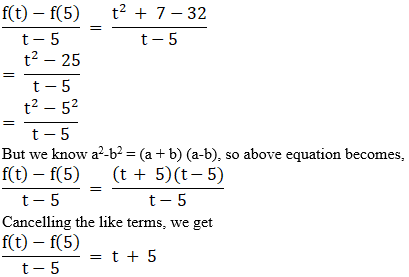12. Let f and g be real functions defined by f (x) = 2x + 1 and g (x) = 4x – 7.
(a) For what real numbers x, f (x) = g (x)?

(b) For what real numbers x, f (x) < g (x)?

Solution:

According to the question,

f and g be real functions defined by f(x) = 2x + 1 and g(x) = 4x – 7

(a) For what real numbers x, f (x) = g (x)

To satisfy the condition f(x) = g(x),

Should also satisfy,

2x + 1 = 4x–7

⇒ 7 + 1 = 4x–2x

⇒ 8 = 2x

Or, 2x = 8

⇒ x = 4

Hence, we get,

For x = 4, f (x) = g (x)

(b) For what real numbers x, f (x) < g (x)

To satisfy the condition f(x) < g(x),

Should also satisfy,

2x + 1 < 4x–7

⇒ 7 + 1 < 4x–2x

⇒ 8 < 2x

Or, 2x > 8

⇒ x > 4

Hence, we get,

For x > 4, f (x) > g (x)

13. If f and g are two real valued functions defined as f (x) = 2x + 1, g (x) = x2 + 1, then find.

(i) f + g (ii) f – g (iii) fg (iv)f/g

Solution:

According to the question,

f and g be real valued functions defined as f (x) = 2x + 1, g (x) = x2 + 1,

(i) f + g

⇒ f + g = f(x) + g(x)

= 2x + 1 + x2 + 1

= x2 + 2x + 2

(ii) f – g

⇒ f – g = f(x) – g(x)

= 2x + 1 – (x2 + 1)

= 2x – x2

(iii) fg

⇒ fg = f(x) g(x)

= (2x + 1)( x2 + 1)

= 2x(x2 ) + 2x(1) + 1(x2) + 1(1)

= 2x3 + 2x + x2 + 1

= 2x3 + x2 + 2x + 1

(iv) f/g

f/g = f(x)/g(x)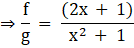14. Express the following functions as set of ordered pairs and determine their range.

f: X → R, f (x) = x3 + 1, where X = {–1, 0, 3, 9, 7}

Solution:

According to the question,

A function f: X →R, f (x) = x3 + 1, where X = {–1, 0, 3, 9, 7}

Domain = f is a function such that the first elements of all the ordered pair belong to the set X = {–1, 0, 3, 9, 7}.

The second element of all the ordered pair are such that they satisfy the condition f (x) = x3 + 1

When x = – 1,

f (x) = x3 + 1

f (– 1) = (– 1)3 + 1 = – 1 + 1 = 0 ⇒ ordered pair = (–1, 0)

When x = 0,

f (x) = x3 + 1

f (0) = (0)3 + 1 = 0 + 1 = 1⇒ ordered pair = (0, 1)

When x = 3,

f (x) = x3 + 1

f (3) = (3)3 + 1 = 27 + 1 = 28⇒ ordered pair = (3, 28)

When x = 9,

f (x) = x3 + 1

f (9) = (9)3 + 1 = 729 + 1 = 730⇒ ordered pair = (9, 730)

When x = 7,

f (x) = x3 + 1

f (7) = (7)3 + 1 = 343 + 1 = 344⇒ ordered pair = (7, 344)

Therefore, the given function as a set of ordered pairs is

f = {(–1, 0), (0, 1), (3, 28), (7, 344), (9, 730)}

And,

Range of f = {0, 1, 28, 730, 344}

15. Find the values of x for which the functions

f (x) = 3x2 – 1 and g (x) = 3 + x are equal

Solution:

According to the question,

f and g functions defined by f (x) = 3x2 – 1 and g (x) = 3 + x

For what real numbers x, f (x) = g (x)

To satisfy the condition f(x) = g(x),

Should also satisfy,

3x2 – 1 = 3 + x

⇒ 3x2 – x – 3 – 1 = 0

⇒ 3x2 – x – 4 = 0

Splitting the middle term,

We get,

⇒ 3x2 + 3x – 4x–4 = 0

⇒ 3x(x + 1) – 4(x + 1) = 0

⇒ (3x – 4)(x + 1) = 0

⇒ 3x – 4 = 0 or x + 1 = 0

⇒ 3x = 4 or x = –1

⇒ x = 4/3, –1

Hence, for x = 4/3, –1, f (x) = g (x),

i.e., given functions are equal.

16. Is g = {(1, 1), (2, 3), (3, 5), (4, 7)} a function? Justify. If this is described by the relation, g (x) = αx + β, then what values should be assigned to α and β?

Solution:

According to the question,

g = {(1, 1), (2, 3), (3, 5), (4, 7)}, and is described by relation g (x) = αx + β

Now, given the relation,

g = {(1, 1), (2, 3), (3, 5), (4, 7)}

g (x) = αx + β

For ordered pair (1,1), g (x) = αx + β, becomes

g (1) = α(1) + β = 1

⇒ α + β = 1

⇒ α = 1 – β …(i)

Considering ordered pair (2, 3), g (x) = αx + β, becomes

g (2) = α(2) + β = 3

⇒ 2α + β = 3

Substituting value of α from equation (i), we get

⇒ 2(2) + β = 3

⇒ β = 3 – 4 = – 1

Substituting value of β in equation (i), we get

α = 1–β = 1–(–1) = 2

Now, the given equation becomes,

i.e., g (x) = 2x–1

17. Find the domain of each of the following functions given by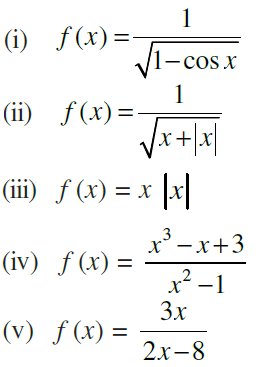Solution:

(i)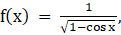According to the question,

We know the value of cos x lies between –1, 1,

–1 ≤ cos x ≤ 1

Multiplying by negative sign, we get

Or 1 ≥ – cos x ≥ –1

2 ≥ 1– cos x ≥ 0 …(i)

Now,1– cos x ≠ 0

⇒ cos x ≠ 1

Or, x ≠ 2nπ ∀ n ∈ Z

Therefore, the domain of f = R–{2nπ:n∈Z}

(ii)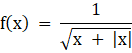According to the question,

For real value of f,

x + |x| > 0

When x > 0,

x + |x| > 0⇒ x + x > 0 ⇒ 2x > 0⇒ x > 0

When x < 0,

x + |x| > 0⇒ x – x > 0 ⇒ 2x > 0⇒ x > 0

So, x > 0, to satisfy the given equation.

Therefore, the domain of f = R+

(iii)

f(x) = x|x|

According to the question,

We know x and |x| are defined for all real values.

Therefore, the domain of f = R

(iv)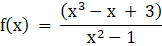According to the question,

For real value of

x2–1≠0

⇒ (x–1)(x + 1)≠0

⇒ x–1≠0 or x + 1≠0

⇒ x≠1 or x≠–1

Therefore, the domain of f = R–{–1, 1}

(v)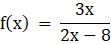According to the question,

For real value of

28 – x ≠0

⇒ x≠ 28

Therefore, the domain of f = R–{28}

18. Find the range of the following functions given by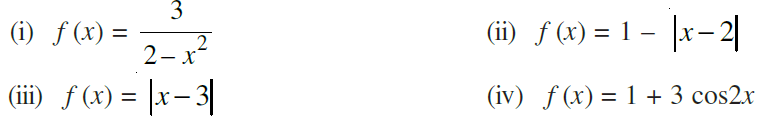Solution:

(i)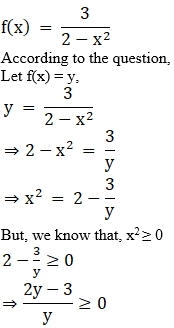⇒ y>0 and 2y–3≥0

⇒ y>0 and 2y≥3

⇒ y>0 and y ≥ 3/2

Or f(x)>0 and f(x) ≥ 3/2

f(x) ∈ ( – ∞, 0) ∪ [ 3/2 , ∞)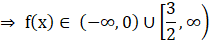Therefore, the range of f = ( – ∞, 0) ∪ [ 3/2 , ∞)

(ii) f(x) = 1–|x–2|

According to the question,

For real value of f,

|x–2|≥ 0

Or –|x–2|≤ 0

⇒ 1–|x–2|≤ 1

Or f(x)≤1

⇒ f(x)∈ (–∞, 1]

Therefore, the range of f = (–∞, 1]

(iii) f(x) = |x–3|

According to the question,

We know |x| are defined for all real values.

And |x–3| will always be greater than or equal to 0.

i.e., f(x) ≥ 0

Therefore, the range of f = [0, ∞)

(iv) f (x) = 1 + 3 cos2x

According to the question,

We know the value of cos 2x lies between –1, 1, so

–1≤ cos 2x≤ 1

Multiplying by 3, we get

–3≤ 3cos 2x≤ 3

–2≤ 1 + 3cos 2x≤ 4

Or, –2≤ f(x)≤ 4

Hence f(x)∈ [–2, 4]

Therefore, the range of f = [–2, 4]

19. Redefine the function f(x) = |x – 2| + |2 + x|, – 3 ≤ x ≤ 3

Solution:

According to the question,

function f(x) = |x–2| + |2 + x|, –3≤ x≤ 3

We know that,

when x>0,

|x – 2| is (x–2), x≥2

|2 + x| is (2 + x), x≥–2

when x>0

|x – 2| is –(x–2), x<2

|2 + x| is –(2 + x), x<–2

Given that, f(x) = |x–2| + |2 + x|, –3≤ x≤ 3

It can be rewritten as,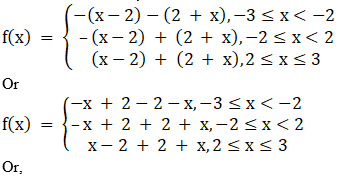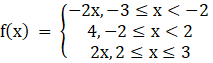20. If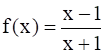, then show that: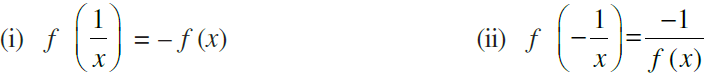Solution:

(i)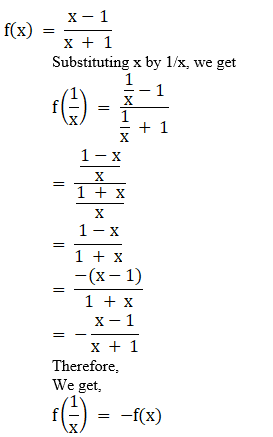Hence proved

(ii)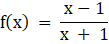Substituting x by – 1/x, we get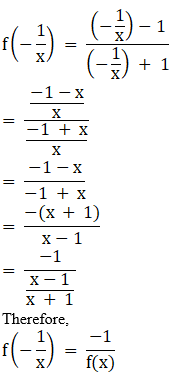Hence proved

21. Let f(x) = √x and g (x) = x be two functions defined in the domain R+∪ {0}. Find

(i) (f + g) (x)

(ii) (f – g) (x)

(iii) (fg) (x)

(iv) (f/g) (x)

Solution:

(i)

(f + g)(x)

⇒ (f + g)(x) = f(x) + g(x)

⇒ f(x) + g(x) = √x + x

(ii)

(f – g)(x)

⇒ (f – g)(x) = f(x) – g(x)

⇒ f(x) – g(x) = √x–x

(iii)

(fg)(x)

⇒ (fg)(x) = f(x) g(x)

⇒ (fg)(x) = (√x)(x)

⇒ f(x)g(x)= x√x

(iv)

(f/q)(x) = f(x)/g(x)